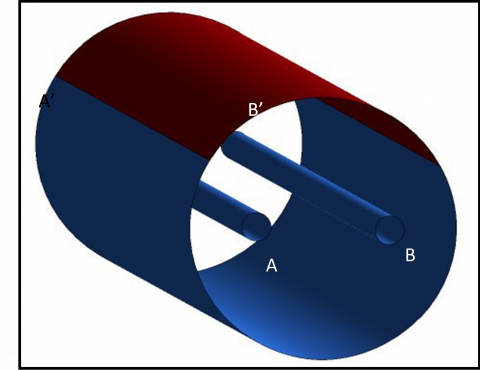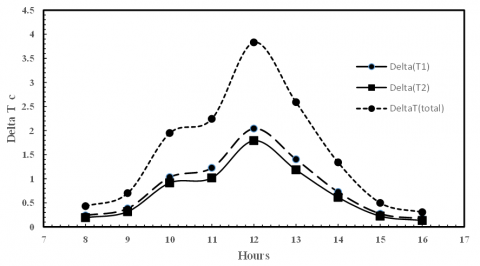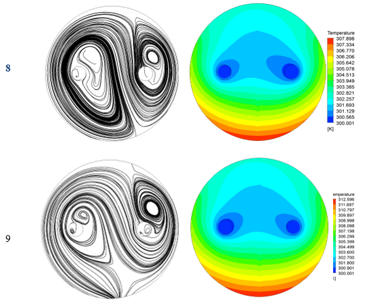# Numerical investigation on effect of Nano-fluid on compound parabolic solar collector performance-Part Ӏ: Time effect

Numerical investigation on effect of Nano-fluid on compound parabolic solar collector performance-Part Ӏ: Time effect

Department of Mechanical Engineering, Urmia University of Technology, Urmia, Iran

Corresponding Author Email:
Page:
10-16
|
DOI:
https://doi.org/10.18280/psees.010102
7 August 2017
| |
Accepted:
8 September 2017
| | Citation

OPEN ACCESS

Abstract:

This research concentrates on heat transfer process in a modern compound parabolic collector (CPC) equipped with two reciprocating cold and hot tubes using Al2O3 as nano particle in the middle fluid (water as the base fluid). The numerical calculations are carried out by the Finite Volume Method applying first order upwind scheme, SIMPLE algorithm and the steady state situation. As the first case, the effect of hours (time) on the heat transfer inside the middle pipes has been analyzed during a complete day (June 22st in Urmia, Iran) assuming a constant volume fraction for nano particles (2%). The results show that the maximum heat transfer occurs in 12 O’clock based on the maximum temperature difference between the inlet and outlet lines of the CPC.

Keywords:

heat transfer, CPC, solar energy, nano particle

1. Introduction

In the last decade, the usage of renewable, clean and free energies (like solar energy) has progressed extremely, because of shortage and pollution of the fossil fuels, also the high price of these types of energy resources is one of the most important limitations in usage of these fuels. The Compound Parabolic Concentrators or CPCs are the attractive and efficient devices which turn the solar radiations to heating or cooling energy. Usually, applying the nano particles in a Newtonian fluid can improve the thermal conductivity of the mixture, so, the heat transfer process between the fluids layers will be done easily compared to systems without nano particles. In this study, Al2O3 nanoparticles have been used within a framework which includes an outer and two inner tubes. In this simulation and analysis, the fluid flow is assumed as a laminar flow (in both inner and outer tubes). Eastman et al.  performed a valuable work on thermal conductivity of fluids (with and without nano particles). Palm et al.  focused on the thermal performance of a cooling system using nanoparticles under forced convection conditions. They found that the nano fluid based on Al2O3 with volume fraction of %4 can increase the heat transfer process upto % 20 compared to pure water. Heris et al.  performed an experimental study on effect of nanoparticles on heat transfer inside the circular tubes using Al2O3. They claimed that when the nanoparticles density is raised the Nusselt number is improved. In 2014, Moghadam et al.  used CuO as a nano particle in water for operating a flat-plate solar collector. They reported that the efficiency of system will be increased by 21.8 % compared to the pure water. Zamzamian et al.  applied Cu-synthesized/EG nanofluid to manage a flat-plate solar collector operating with Cu-synthesized/EG nanofluid. They observed that when the volume fraction of the  nanoparticle is increased, the thermal effectiveness of the system is raised. The theoretical studies  on nanoparticles effect revealed that TiO2, Al2O3, SWCNT, SiO2 decrease the entropy production around 4.34% and increase the heat transmission factor around 15.33%. This study focuses on effect of nanoparticles in middle fluid on thermal performance of a determined CPC during a complete day. This effect on the CPC performance is not analyzed yet, and this project tries to extend the observations on this subject.

2. Geometry Definition and Boundary Conditions

Fig. 1 shows a schematic model with the important geometrical parameters like inlets and outlets.

## 1.pngFigure 1. Compound Parabolic Collector (CPC) with two middle pipes including inlet and outlet

In this simulation, the covering pipe (main solar collector) contains the mixture of nanoparticles and water as the middle fluid. There are two other small pipes, one is the cold water or input line (AA’) and the other is the hot water or output line (BB’). The hot water or output line (B’) and the cold water or input line (A’) are connected in the simulation via a UDF code in FLUENT software. The top surface of the collector wall is the direct receiver surface and q" or the heat flux is received (directly) from the sun on this surface which is called $W A L L_{S}$ and the bottom part of the collector (large pipe) receives 5q" heat flux from the parabolic reflection which is called ($W A L L_{C}$). The diameter of internal and external pipes (middle and main collector) also the length of the main domain are considered 6, 55 and 500 mm, respectively. The assumptions are as bellow:

Inlet $\mathrm{A}\left(u=0, v=0, w=w_{0}, T=T_{i n}\right)$    (1)

Outlet A’ and inlet B’$\left(\frac{\partial w}{\partial z}=0, \frac{\partial T}{\partial z}=0, p=0\right.$ and $u=0, v=0, w=-w_{A^{\prime}} )$    (2)

Inlet Nano fluid $\left(\text {wall slip, } \frac{\partial T}{\partial n}=\mathbf{0}\right)$    (3)

Outlet Nano fluid $\left(\text {wall slip, } \frac{\partial T}{\partial n}=\mathbf{0}\right)$    (4)

Walls of AA’ and BB’(temperature copled, no -slip u=v=w=0)

(5)

$W a l l_{s}\left(\frac{\partial T}{\partial n}=q^{\prime \prime}, u=v=w=0\right)$    (6)

$W a l l_{c}\left(\frac{\partial T}{\partial n}=5 q^{\prime \prime}, u=v=w=0\right)$    (7)

3. Mathematical Modeling and Numerical Investigation

The governing equations for the three-dimensional and laminar flow are written as:

Continuity equation

$\frac{\partial u}{\partial x}+\frac{\partial v}{\partial y}+\frac{\partial w}{\partial z}=0$    (8)

Momentum equation

$\rho_{n f}\left(u \frac{\partial u}{\partial x}+v \frac{\partial u}{\partial y}+w \frac{\partial u}{\partial z}\right)=-\frac{\partial p}{\partial x}+\mu_{n f}\left(\frac{\partial^{2} u}{\partial x^{2}}+\frac{\partial^{2} u}{\partial y^{2}}+\frac{\partial^{2} u}{\partial z^{2}}\right)$    (9)

$\rho_{n f}\left(u \frac{\partial v}{\partial x}+v \frac{\partial v}{\partial y}+w \frac{\partial v}{\partial z}\right)=-\frac{\partial p}{\partial y}+\mu_{n f}\left(\frac{\partial^{2} v}{\partial x^{2}}+\frac{\partial^{2} v}{\partial y^{2}}+\frac{\partial^{2} v}{\partial z^{2}}\right)+\rho_{n f} \beta_{n f}\left(T-T_{r e f}\right)$    (10)

$\rho_{n f}\left(u \frac{\partial w}{\partial x}+v \frac{\partial w}{\partial y}+w \frac{\partial w}{\partial z}\right)=-\frac{\partial p}{\partial z}+\mu_{n f}\left(\frac{\partial^{2} w}{\partial x^{2}}+\frac{\partial^{2} w}{\partial y^{2}}+\frac{\partial^{2} w}{\partial z^{2}}\right)$    (11)

Energy equation

$\left(\rho c_{p}\right)_{n f}\left(u \frac{\partial T}{\partial x}+v \frac{\partial T}{\partial y}+w \frac{\partial T}{\partial z}\right)=\mathrm{K}_{n f}\left(\frac{\partial^{2} T}{\partial x^{2}}+\frac{\partial^{2} T}{\partial y^{2}}+\frac{\partial^{2} T}{\partial z^{2}}\right)$    (12)

$\frac{\overline{I}}{\overline{I_{0}}}=a+b\left(\frac{\overline{n}}{\overline{N}}\right)$    (13)

where, a,b are two constant values, for Urmia are equal to 0.402 and 0.305, respectively.

$\overline{N}=\frac{2}{15} \omega_{S}$    (14)

$G_{S C}$ is the radiation received per square meter from the atmosphere, $G_{S C}$=1373. Also, $G_{S}$ is calculated based on the day number and the solar constant $G_{S C}$, and $\overline{H}_{o}$ is the daily radiation module for the outer space:

$G_{s}=G_{s c} \times\left(1+0.033 \times \cos \left(\frac{360 n}{365}\right)\right)$    (15)

$\overline{H}_{o}=24 \times \frac{3600}{\pi} G_{S} \times\left[\cos \varphi \cos \delta \sin \omega_{S}+\frac{\pi \omega s}{180} \sin \varphi \cos \varphi\right]$    (16)

where, $\delta$ is the consider angle between a line that connects two centers of the earth and the sun and the equator plane which is between $-23.45 \leq \delta \leq 23.45$:

$\delta=23.45 \sin \left(360 \frac{284+n}{365}\right)$    (17)

$\omega_{S}$ is the hour angle or the sun’s angular deviation from the south that is considered between $-180 \leq \omega_{s} \leq 180$:

$\omega_{S}=15 \times(12-t)$    (18)

$\omega_{S C}=\cos ^{-1}(-\tan \varphi \tan \delta)$    (19)

$I_{0}$ and $\varphi$ are the radiation hourly module and the location altitude, respectively :

$\overline{I_{o}}=24 \times \frac{3600}{\pi} G_{s} \times\left[\cos \varphi \cos \delta \sin \left(\sin \omega_{2}-\sin \omega_{1}\right)+\frac{\pi\left(\omega_{2}-\omega_{1}\right)}{180} \sin \varphi \cos \delta\right]$    (20)

The heat capacitance and the effective density of the nano fluid are given as:

$\rho_{n f}=(1-\phi) \rho_{b f}+\phi \rho_{P}$    (21)

$\left(\rho C_{p}\right)_{n f}=(1-\phi)\left(\rho C_{p}\right)_{b f}+\phi\left(\rho C_{p}\right)_{p}$    (22)

The effective thermal conductivity of the nano fluid is given by:

$k_{n f}=\frac{k_{p}+2 k_{f}-2 \phi\left(k_{f}-k_{p}\right)}{k_{p}+2 k_{f}+\phi\left(k_{f}-k_{p}\right)} k_{f}$        (23)

The dynamic viscosity and the thermal expansion coefficient of the solid-liquid mixture can be defined by:

$\mu_{n f}=\frac{\mu_{b f}}{(1-\phi)^{2.5}}$    (24)

$\beta_{n f}=(1-\phi) \beta_{b f}+\phi \beta_{P}$    (25)

And the absorption coefficient of the nano fluid is:

$\alpha_{n f}=\frac{k_{n f}}{\left(\rho c_{p}\right)_{n f}}$    (26)

The above equations can be introduced by the following non-dimensional variables:

$Y=\frac{y}{h}, X=\frac{x}{h}, P=\frac{p}{\rho_{f}\left(\frac{\alpha f}{l}\right)^{2}}, U=\frac{u l}{\alpha_{f}}, d_{i}=h \partial_{i}, \theta=\frac{T-T_{W}}{T_{F}-T_{W}}, V=\frac{v l}{\alpha_{f}}$    (27)

$\frac{\partial U}{\partial X}+\frac{\partial V}{\partial Y}+\frac{\partial W}{\partial Z}=0$    (28)

$\left(U \frac{\partial U}{\partial X}+V \frac{\partial U}{\partial Y}+W \frac{\partial U}{\partial Z}\right)=-\frac{\partial P}{\partial X}+\operatorname{Pr}\left[\frac{\mu_{n f} / \mu_{f}}{\rho_{n f} / \rho_{f}}\right]\left(\frac{\partial^{2} U}{\partial X^{2}}+\frac{\partial^{2} U}{\partial Y^{2}}+\frac{\partial^{2} U}{\partial Z^{2}}\right)$              (29)

$\left(U \frac{\partial V}{\partial X}+V \frac{\partial V}{\partial Y}+W \frac{\partial V}{\partial Z}\right)$$=-\frac{\partial P}{\partial Y}+\operatorname{Pr}\left[\frac{\mu_{n f} / \mu_{f}}{\rho_{n f} / \rho_{f}}\right]\left(\frac{\partial^{2} V}{\partial X^{2}}+\frac{\partial^{2} V}{\partial Y^{2}}+\frac{\partial^{2} V}{\partial Z^{2}}\right)$$+R a \operatorname{Pr}\left[\beta_{n f} / \beta_{f}\right] \theta$    (30)

$\left(U \frac{\partial W}{\partial X}+V \frac{\partial W}{\partial Y}+W \frac{\partial W}{\partial Z}\right)=-\frac{\partial P}{\partial Z}+\operatorname{Pr}\left[\frac{\mu_{n f} / \mu_{f}}{\rho_{n f} / \rho_{f}}\right]\left(\frac{\partial^{2} W}{\partial X^{2}}+\frac{\partial^{2} W}{\partial Y^{2}}+\frac{\partial^{2} W}{\partial Z^{2}}\right)$    (31)

$\left(U \frac{\partial \theta}{\partial X}+V \frac{\partial \theta}{\partial Y}+W \frac{\partial \theta}{\partial Z}\right)=\left[\frac{k_{n f} / k_{f}}{(\rho c p)_{n f} / (\rho c p)_{f}}\right]\left(\frac{\partial^{2} \theta}{\partial X^{2}}+\frac{\partial^{2} \theta}{\partial Y^{2}}+\frac{\partial^{2} \theta}{\partial Z^{2}}\right)+E c\left[\frac{\mu_{n f} / \mu_{f}}{(\rho c p)_{n f} / (\rho c p)_{f}}\right]$                        (32)

where, $P_{r}=\vartheta_{f} / \alpha_{f}$, $E c=\left(\mu_{f} \alpha_{f} /\left[\left(\rho C_{p}\right)_{f} \Delta T L^{2}\right]\right)$, Ra = $\left(g \beta_{f} q^{\prime \prime} L^{4} / \vartheta_{f} \alpha_{f} k\right)$, $\varnothing$ are the Prandtl number, the Eckert number, the Rayleigh number and the volume fraction of the nanoparticle, respectively. The thermophysical properties of the mentioned nano fluids are presented in Table 1.

Table 1. Thermo physical properties of the solid-liquid mixture

 $\rho\left(k g / m^{3}\right)$ $c_{p}(j / k g k)$ $k(w / m k)$ $\beta* 10^{-5}\left(k^{-1}\right)$ Water pure 998.2 4179 0.613 21 Al2O3 3970 765 40 1.3 TiO2 4230 686.2 8.95 24

Fig. 2 shows the mesh domain in a sectional surface and the mesh independence study based on 44000, 220000 and 440000 cells.

## 2a.png## 2b-.pngFigure 2. Grid independence study and the related mesh domain

4. Results

In Table 2 the heat flux changes and the equivalent Rayleigh number has been provided for different hours in June 22st.

Table 2. Heat flux and the equivalent Rayleigh number for different hours in June 22st

 clock 8 9 10 11 12 13 14 15 16 $q^{\prime \prime}(w / m^{2})$ 35.604 53.497 86.363 178.332 183.592 168.856 77.272 45.027 27.956 $R a \times 10^{7}$ 2.84 4.27 6.89 14.2 14.6 13.5 6.16 3.59 2.23

The boundary condition for the collector wall (both top and bottom) is fixed as the heat flux condition equal to $q^{\prime \prime}$ and 5$q^{\prime \prime}$, respectively.

The equivalent Rayleigh number is calculated by Ra=$\left(\mathrm{g} \beta_{\mathrm{f}} \mathrm{q}_{\mathrm{ref}}^{\prime \prime} \mathrm{L}^{4} / \vartheta_{\mathrm{f}} \alpha_{\mathrm{f}} \mathrm{k}\right)$, in which, $q_{\text { ref }}^{\prime \prime}$ is the average amount of the heat flux between the sheets.

Fig. 3 shows the variations of input and output temperatures in both pipes (as seen in Fig. 1), $\Delta T_{1}=T_{A^{\prime}}-T_{A}$, $\Delta T_{2}=T_{B}-T_{B^{\prime}}$, $\Delta T_{t}=T_{B}-T_{A}$. According to Fig. 3, the highest temperature difference value is 3.83 ℃ at 12 O'clock. Also, when the thermal flux is increased gradually, the temperature difference or the water warming rate increases.

## qqtu_pian_20190710160501.pngFigure 3. Input and output temperature changes in both pipes

Time

Stream lines

Temperature contour

## 1.png## 4-2.png## 134.png## 33.pngFigure 4. Total temperature contours and streamlines in different hours

Fig. 4 shows the stream lines and the temperature contours for the pipe surface at Z=0.25 for different times. The left pipe is the cold water or entering line into the system and is installed vertically. The pipe on the right side is the warmed water obtained from the system leading out of direction of the sheets. According to Fig. 4, the vortex around the cold line is bigger than the vortex around the hot tube. This event is because of the temperature difference between the cold and the hot regions. In other words, the temperature difference between the bottom wall and the cold pipe is higher than the temperature difference between the bottom wall and the hot pipe. According to Fig. 4, when the heat flux increases (get closer to noon), the temperature difference between the bottom wall and the cold line arises, consequently, the vortex size around the cold pipe becomes bigger.

Fig. 5 shows the velocity profile on the line -0.0275<x < 0.0275, y=0, z=0.25. It should be said that the vortices turning around the water pipes increases the heat transfer rate. It can be seen that (according to the velocity profiles) with increase in the thermo flux value or when the time gets closer to noon the speed of flow is raised and the profile speed shows the highest rate. The elevated flow speed and the free convection (free motion of the flow patterns inside the CPC) increase the heat transfer and the average Nusselt number.

## 5.pngFigure 5. Velocity distribution inside the CPC as a function of tube diameter

5. Conclusions

This paper tries to analyze the heat transfer process in a modern compound parabolic collector (CPC) equipped with two reciprocating cold and hot tubes using Al2O3 as a nano particle in the middle fluid. A three dimensional model is designed based on a real model which is tested successfully.  The boundary condition for the collector walls including both top and bottom surfaces are fixed as the heat flux condition equal to $q^{\prime \prime}$ and 5$q^{\prime \prime}$, respectively. According to the results, the highest temperature difference value is 3.83 ℃ at 12 O'clock. Also, when the thermal flux is increased gradually, the temperature difference or the water warming rate increases. According to the results the vortex around the cold line is bigger than the vortex around the hot tube. This event is because of the temperature difference between the cold and the hot regions. In other words, the temperature difference between the bottom wall and the cold pipe is higher than the temperature difference between the bottom wall and the hot pipe.

Nomenclature

Ec                  Eckert number, $u_{f} \alpha_{f} k /\left[\left(\rho c_{p}\right)_{f} q^{\prime \prime} L^{3}\right]$

CP                 specific heat at constant pressure

Gr                  Grashof number

$N U_{l o}$              local Nusselt number

$N U_{a v e}$          average Nusselt number

Pr                   Prandtl number, $\vartheta_{f} / \alpha_{f}$

T                    fluid temperature

u,v,w               velocity component in the x-direction and y-direction and z-direction

X,Y,Z               dimensionless space coordinates

U,V,W             dimensionless velocity components in the X-direction and Y-direction and Z-direction

K                    thermal conductivity

Ra                  Rayleigh Number $\left(=g \beta_{f} q^{\prime \prime} L^{4} / k \alpha_{f} \vartheta_{f}\right)$

Greek symbols

$\alpha$                     thermal diffusivity

$\vartheta$                     kinematic viscosity

$\emptyset$                    volume fraction

$\mu$                     dynamic viscosity

$\theta$                     dimensionless temperature

$\rho$                     fluid density

$\beta$                     thermal expansion coefficient

Subscripts

c                      cold

h                      hot

nf                     nanofluid

f                       base fluid

n                      nanoparticle

in                     inlet

out                   outlet

References

 Eastman JA, Choi US, Li S, Thompson LJ, Lee S. (1996). Enhanced thermal conductivity through the development of nanofluids. MRS Proceedings 457: 3.

 Palm SJ, Roy G, Nguyen CT. (2006). Heat transfer enhancement with the use of nanofluids in radial flow cooling systems considering temperature-dependent properties. Applied Thermal Engineering 26(17): 2209-2218.

 Heris SZ, Esfahany MN, Etemad SG. (2007). Experimental investigation of convective heat transfer of Al2O3/water nanofluid in circular tube. International Journal of Heat and Fluid Flow 28(2): 203-210.

 Moghadam AJ, Farzane-Gord M, Sajadi M, Hoseyn-Zadeh M. (2014). Effects of CuO/water nanofluid on the efficiency of a flat-plate solar collector. Experimental Thermal and Fluid Science 58: 9-14.

 Zamzamian A, KeyanpourRad M, KianiNeyestani M, Jamal-Abad MT. (2014). An experimental study on the effect of Cu-synthesized/EG nanofluid on the efficiency of flat-plate solar collectors. Renewable Energy 71: 658-664.

 Mahian O, Kianifar A, Zeinali Heris S, Wongwises S. (2014). First and second laws analysis of a minichannel-based solar collector using boehmite alumina nanofluids: Effects of nanoparticle shape and tube materials. International Journal of Heat and Mass Transfer 78: 1166-1176.